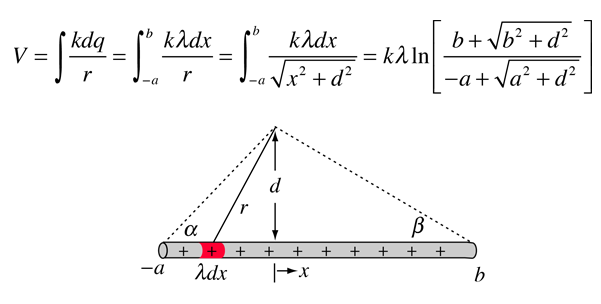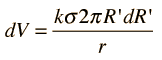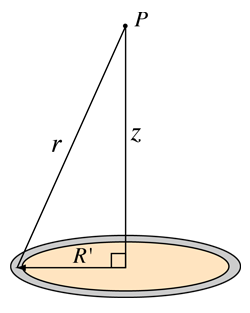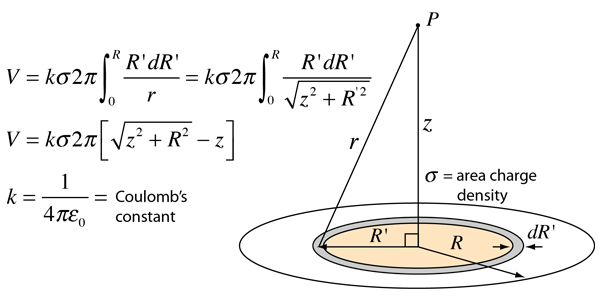# Potential of Line Charge

The potential of a line of charge can be found by superposing the point charge potentials of infinitesmal charge elements. It is an example of a continuous charge distribution.Electric field of finite line charge
 Potentials for other charge geometries
Index

Voltage concepts

 HyperPhysics***** Electricity and Magnetism R Nave
Go Back

# Potential for Ring of Charge

The potential of a ring of charge can be found by superposing the point charge potentials of infinitesmal charge elements. It is an example of a continuous charge distribution. The ring potential can then be used as a charge element to calculate the potential of a charged disc.

 Since the potential is a scalar quantity, and since each element of the ring is the same distance r from the point P, the potential is simply given byIf the charge is characterized by an area density and the ring by an incremental width dR', then:In this form it could be used as a charge element for the determining of the potential of a disc of charge.

 Electric field of a ring of charge
 Potentials for other charge geometries
Index

Voltage concepts

 HyperPhysics***** Electricity and Magnetism R Nave
Go Back

# Potential for Disc of Charge

The potential of a disc of charge can be found by superposing the point charge potentials of infinitesmal charge elements. It is an example of a continuous charge distribution. The evaluation of the potential can be facilitated by summing the potentials of charged rings. The integral over the charged disc takes the form:Electric field of disc of charge
 Potentials for other charge geometries
Index

Voltage concepts

 HyperPhysics***** Electricity and Magnetism R Nave
Go Back